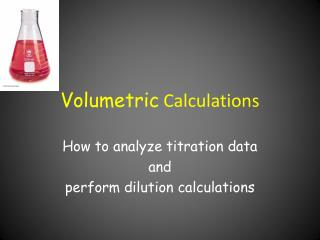DownloadDownload PresentationVolumetric Calculations

# Volumetric Calculations

Télécharger la présentation## Volumetric Calculations

- - - - - - - - - - - - - - - - - - - - - - - - - - - E N D - - - - - - - - - - - - - - - - - - - - - - - - - - -
##### Presentation Transcript

1. Volumetric Calculations How to analyze titration data and perform dilution calculations

2. Volumetric procedures • Involve the careful measurement of volumes of solutions • Dissolve materials in water and run reaction in solution - titration in Erlenmeyer flask (designed for swirling to mix reactants) • Buret is the measuring device - can read volumes to 0.01 mL • Pipets may also be used, especially to transfer solutions - good to 0.01 mL

3. Some terminology • Analyte • Titrant • Standard solution • Equivalence point • End point Substance being analyzed Solution in the buret Solution of known concentration Stoichiometric point

4. At the equivalence point • For a 1-to-1 reaction such as: HCl + NaOH  NaCl + H2O mole acid = mole base • moles = molarity x volume in liters • So Macid x Vacid = Mbase x Vbase

5. What is the end point? • The end point is the point where an indicator changes color. • This is the visual sign to stop or end the titration. If the indicator is selected correctly, the change is at or very near the equivalence point! • Example: phenolphthalein colorless in acid pinkin base

6. Example of Concentration Calculation • A 25.07 mL sample of vinegar is titrated with 37.31 mL of 0.5119 M NaOH. What is the molarity of the acetic acid in vinegar? Moles NaOH = 0.5119 moles/L x 37.31 mL x 1L/1000mL Moles acetic acid = moles NaOH = 0.01910 moles Maa = moles/volume = 0.01910 moles/0.02507 L = 0.7618 M

7. Calculation of Analyte Mass • A 11.50 g sample of lemon juice is titrated with 0.202M NaOH to determine the mass of the citric acid, a triprotic acid. If it took 36.48 mL of base, what is the mass of C6H8O7? What is the % citric acid by mass? • C6H8O7 + 3 NaOH Na3C6H5O7 + 3 H2O • Mb x Vb = molb 0.202 M x 0.03648 L = 0.00737 mol

8. Analyte Mass Continued • molb/3 = mola 0.00737/3 = 0.00246 mol • mola x MMa = ga 0.00246 mol x 192.1 g/mol = 0.473 g C6H8O7 • % = (mass C6H8O7/mass sample) x 100 • % = (0.473/11.50) x 100 = 4.11 % C6H8O7

9. Back Titration • An indirect analysis for antacids, since they are insoluble • Antacids, which are bases, are reacted with excess acid and this excess acid is titrated with standard base. Antacid + HCl (initial)  neutralized antacid + HCl (leftover) HCl (leftover) + NaOH  NaCl + H2O

10. Back Titration Calculation- • moles HCl initially = MHCl x VHCl • moles HCl left-over = moles NaOH = MNaOH x VNaOH • mole HCl reacted = moles HCl initially - moles HCl left-over • moles antacids - depends on reaction stoichiometry: CaCO3 + 2HCl  CaCl2 + H2O + CO2

11. Dilution • Process of adding solvent, usually water, to lower the concentration of a solution • Used to make working standards for spectrophotometry • Used to bring a sample into range for analysis • Typically done using a pipet (TD) and volumetric flask (TC) Solute is constant. Solvent is being added.

12. Dilution continued • Since the solute is constant, then molesinitial = molesfinal • So the dilution equation becomes Cinit Vinit = Cfinal Vfinal where Vfinal = Vinit + Vwater added

13. Dilution Calculation Cinit Vfinal • What initial volume of 0.50 M CuSO4 must be used to make 100 mL of 0.025 M CuSO4 solution? • Cinit Vinit = Cfinal Vfinal and rearrange • Vinit = Cfinal Vfinal / Cinit =0.025 M x 100 mL/ 0.50 M = 5.0 mL Cfinal

14. Dilution continued • A graduated cylinder is a volume measuring device to contain (TC). Could you do a dilution in it? Explain why or why not. • Dilution factor = Vinit/Vfinal 1 mL into 100 mL is a df of 1/100

15. Dilution continued • Serial dilution – successive dilutions for large scale dilutions performed in steps A is diluted by 1/100 to B B is diluted by 1/100 to C C is 1/10,000 the concentration of A